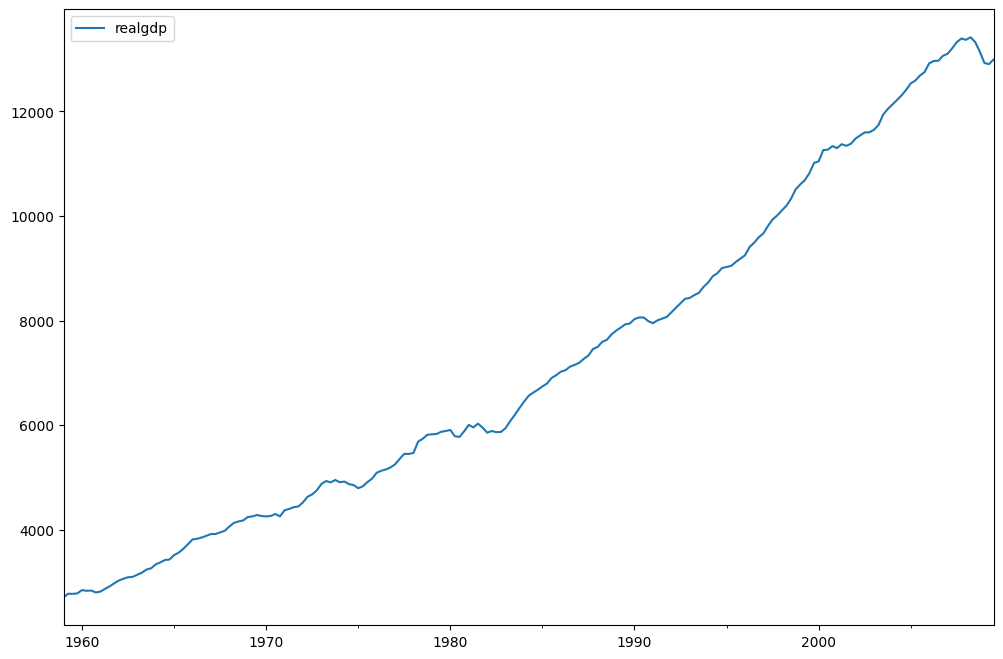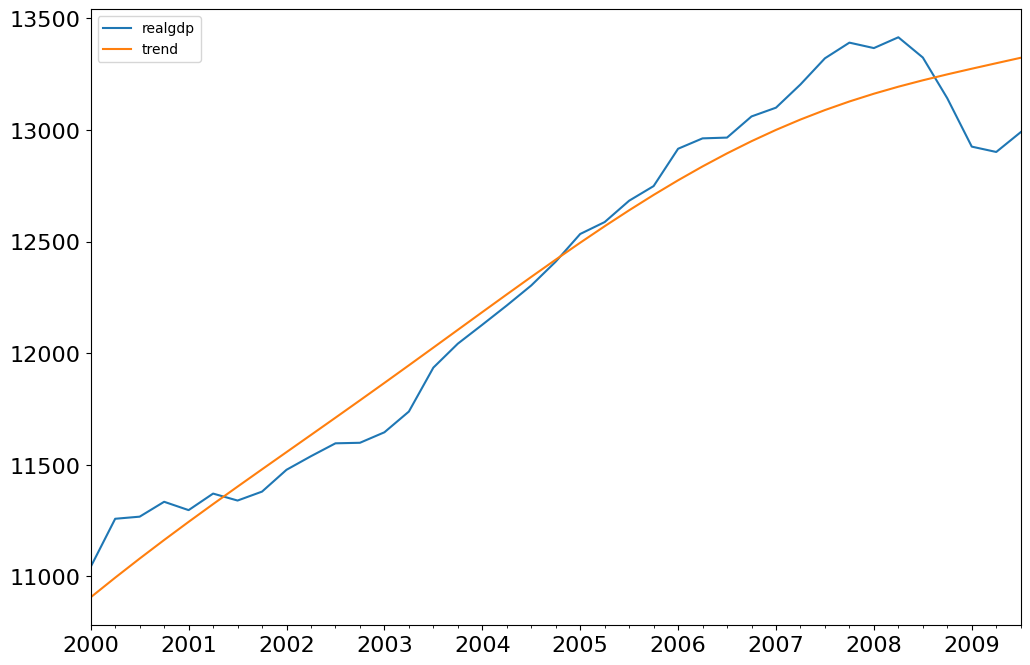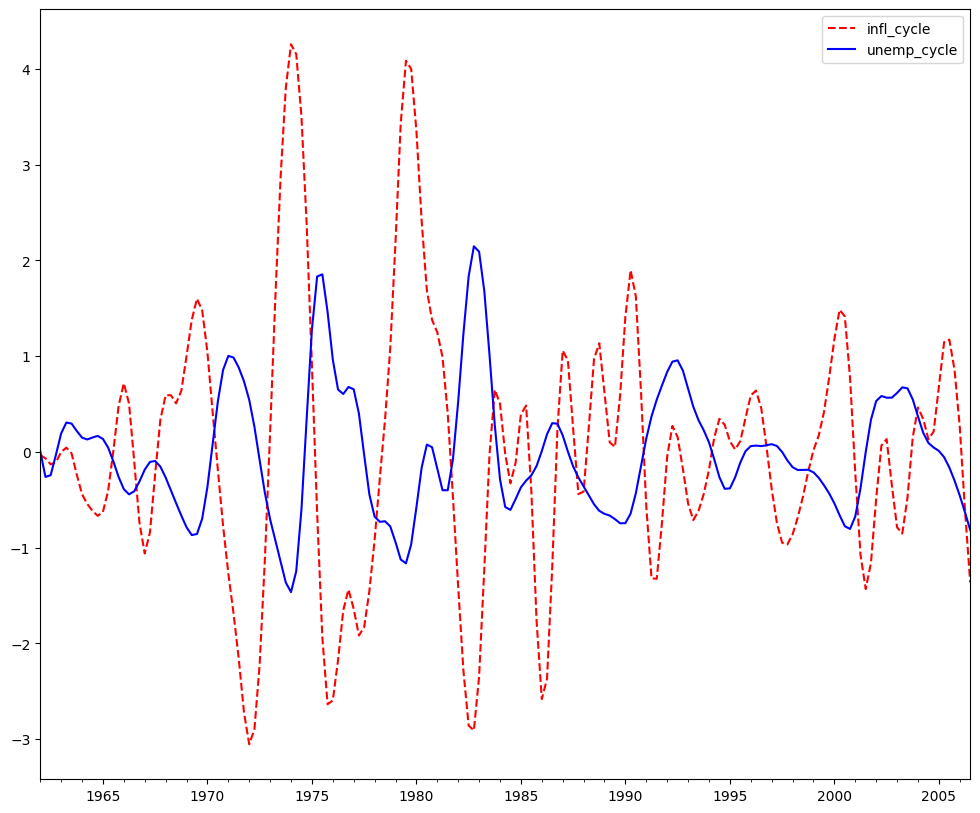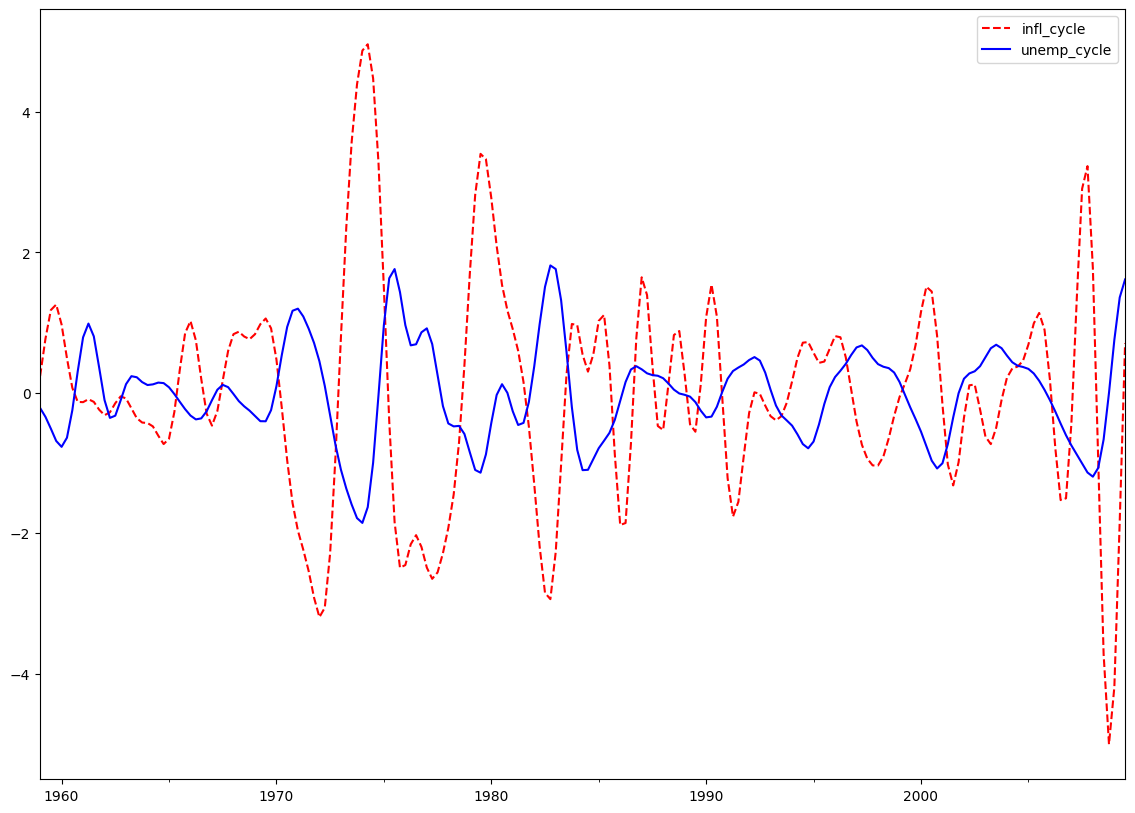# Time Series Filters¶

:

%matplotlib inline

:

import pandas as pd
import matplotlib.pyplot as plt

import statsmodels.api as sm

:

dta = sm.datasets.macrodata.load_pandas().data

:

index = pd.Index(sm.tsa.datetools.dates_from_range("1959Q1", "2009Q3"))
print(index)

DatetimeIndex(['1959-03-31', '1959-06-30', '1959-09-30', '1959-12-31',
'1960-03-31', '1960-06-30', '1960-09-30', '1960-12-31',
'1961-03-31', '1961-06-30',
...
'2007-06-30', '2007-09-30', '2007-12-31', '2008-03-31',
'2008-06-30', '2008-09-30', '2008-12-31', '2009-03-31',
'2009-06-30', '2009-09-30'],
dtype='datetime64[ns]', length=203, freq=None)

:

dta.index = index
del dta["year"]
del dta["quarter"]

:

print(sm.datasets.macrodata.NOTE)

::
Number of Observations - 203

Number of Variables - 14

Variable name definitions::

year      - 1959q1 - 2009q3
quarter   - 1-4
realgdp   - Real gross domestic product (Bil. of chained 2005 US$, seasonally adjusted annual rate) realcons - Real personal consumption expenditures (Bil. of chained 2005 US$, seasonally adjusted annual rate)
realinv   - Real gross private domestic investment (Bil. of chained
2005 US$, seasonally adjusted annual rate) realgovt - Real federal consumption expenditures & gross investment (Bil. of chained 2005 US$, seasonally adjusted annual rate)
realdpi   - Real private disposable income (Bil. of chained 2005
cpi       - End of the quarter consumer price index for all urban
consumers: all items (1982-84 = 100, seasonally adjusted).
m1        - End of the quarter M1 nominal money stock (Seasonally
tbilrate  - Quarterly monthly average of the monthly 3-month
treasury bill: secondary market rate
unemp     - Seasonally adjusted unemployment rate (%)
pop       - End of the quarter total population: all ages incl. armed
forces over seas
infl      - Inflation rate (ln(cpi_{t}/cpi_{t-1}) * 400)
realint   - Real interest rate (tbilrate - infl)


:

print(dta.head(10))

             realgdp  realcons  realinv  realgovt  realdpi    cpi     m1  \
1959-03-31  2710.349    1707.4  286.898   470.045   1886.9  28.98  139.7
1959-06-30  2778.801    1733.7  310.859   481.301   1919.7  29.15  141.7
1959-09-30  2775.488    1751.8  289.226   491.260   1916.4  29.35  140.5
1959-12-31  2785.204    1753.7  299.356   484.052   1931.3  29.37  140.0
1960-03-31  2847.699    1770.5  331.722   462.199   1955.5  29.54  139.6
1960-06-30  2834.390    1792.9  298.152   460.400   1966.1  29.55  140.2
1960-09-30  2839.022    1785.8  296.375   474.676   1967.8  29.75  140.9
1960-12-31  2802.616    1788.2  259.764   476.434   1966.6  29.84  141.1
1961-03-31  2819.264    1787.7  266.405   475.854   1984.5  29.81  142.1
1961-06-30  2872.005    1814.3  286.246   480.328   2014.4  29.92  142.9

tbilrate  unemp      pop  infl  realint
1959-03-31      2.82    5.8  177.146  0.00     0.00
1959-06-30      3.08    5.1  177.830  2.34     0.74
1959-09-30      3.82    5.3  178.657  2.74     1.09
1959-12-31      4.33    5.6  179.386  0.27     4.06
1960-03-31      3.50    5.2  180.007  2.31     1.19
1960-06-30      2.68    5.2  180.671  0.14     2.55
1960-09-30      2.36    5.6  181.528  2.70    -0.34
1960-12-31      2.29    6.3  182.287  1.21     1.08
1961-03-31      2.37    6.8  182.992 -0.40     2.77
1961-06-30      2.29    7.0  183.691  1.47     0.81

:

fig = plt.figure(figsize=(12, 8))
dta.realgdp.plot(ax=ax)
legend = ax.legend(loc="upper left")
legend.prop.set_size(20)## Hodrick-Prescott Filter¶

The Hodrick-Prescott filter separates a time-series $$y_t$$ into a trend $$\tau_t$$ and a cyclical component $$\zeta_t$$

$y_t = \tau_t + \zeta_t$

The components are determined by minimizing the following quadratic loss function

$\begin{split}\min_{\\{ \tau_{t}\\} }\sum_{t}^{T}\zeta_{t}^{2}+\lambda\sum_{t=1}^{T}\left[\left(\tau_{t}-\tau_{t-1}\right)-\left(\tau_{t-1}-\tau_{t-2}\right)\right]^{2}\end{split}$
:

gdp_cycle, gdp_trend = sm.tsa.filters.hpfilter(dta.realgdp)

:

gdp_decomp = dta[["realgdp"]].copy()
gdp_decomp["cycle"] = gdp_cycle
gdp_decomp["trend"] = gdp_trend

:

fig = plt.figure(figsize=(12, 8))
gdp_decomp[["realgdp", "trend"]]["2000-03-31":].plot(ax=ax, fontsize=16)
legend = ax.get_legend()
legend.prop.set_size(20)## Baxter-King approximate band-pass filter: Inflation and Unemployment¶

### Explore the hypothesis that inflation and unemployment are counter-cyclical.¶

The Baxter-King filter is intended to explicitly deal with the periodicity of the business cycle. By applying their band-pass filter to a series, they produce a new series that does not contain fluctuations at higher or lower than those of the business cycle. Specifically, the BK filter takes the form of a symmetric moving average

$y_{t}^{*}=\sum_{k=-K}^{k=K}a_ky_{t-k}$

where $$a_{-k}=a_k$$ and $$\sum_{k=-k}^{K}a_k=0$$ to eliminate any trend in the series and render it stationary if the series is I(1) or I(2).

For completeness, the filter weights are determined as follows

$a_{j} = B_{j}+\theta\text{ for }j=0,\pm1,\pm2,\dots,\pm K$
$B_{0} = \frac{\left(\omega_{2}-\omega_{1}\right)}{\pi}$
$B_{j} = \frac{1}{\pi j}\left(\sin\left(\omega_{2}j\right)-\sin\left(\omega_{1}j\right)\right)\text{ for }j=0,\pm1,\pm2,\dots,\pm K$

where $$\theta$$ is a normalizing constant such that the weights sum to zero.

$\theta=\frac{-\sum_{j=-K^{K}b_{j}}}{2K+1}$
$\omega_{1}=\frac{2\pi}{P_{H}}$
$\omega_{2}=\frac{2\pi}{P_{L}}$

$$P_L$$ and $$P_H$$ are the periodicity of the low and high cut-off frequencies. Following Burns and Mitchell’s work on US business cycles which suggests cycles last from 1.5 to 8 years, we use $$P_L=6$$ and $$P_H=32$$ by default.

:

bk_cycles = sm.tsa.filters.bkfilter(dta[["infl", "unemp"]])

• We lose K observations on both ends. It is suggested to use K=12 for quarterly data.

:

fig = plt.figure(figsize=(12, 10))
bk_cycles.plot(ax=ax, style=["r--", "b-"])

:

<Axes: >## Christiano-Fitzgerald approximate band-pass filter: Inflation and Unemployment¶

The Christiano-Fitzgerald filter is a generalization of BK and can thus also be seen as weighted moving average. However, the CF filter is asymmetric about $$t$$ as well as using the entire series. The implementation of their filter involves the calculations of the weights in

$y_{t}^{*}=B_{0}y_{t}+B_{1}y_{t+1}+\dots+B_{T-1-t}y_{T-1}+\tilde B_{T-t}y_{T}+B_{1}y_{t-1}+\dots+B_{t-2}y_{2}+\tilde B_{t-1}y_{1}$

for $$t=3,4,...,T-2$$, where

$B_{j} = \frac{\sin(jb)-\sin(ja)}{\pi j},j\geq1$
$B_{0} = \frac{b-a}{\pi},a=\frac{2\pi}{P_{u}},b=\frac{2\pi}{P_{L}}$

$$\tilde B_{T-t}$$ and $$\tilde B_{t-1}$$ are linear functions of the $$B_{j}$$’s, and the values for $$t=1,2,T-1,$$ and $$T$$ are also calculated in much the same way. $$P_{U}$$ and $$P_{L}$$ are as described above with the same interpretation.

The CF filter is appropriate for series that may follow a random walk.

:

print(sm.tsa.stattools.adfuller(dta["unemp"])[:3])

(-2.53645846733463, 0.10685366457233608, 9)

:

print(sm.tsa.stattools.adfuller(dta["infl"])[:3])

(-3.054514496257235, 0.030107620863486007, 2)

:

cf_cycles, cf_trend = sm.tsa.filters.cffilter(dta[["infl", "unemp"]])

            infl_cycle  unemp_cycle
1959-03-31    0.237927    -0.216867
1959-06-30    0.770007    -0.343779
1959-09-30    1.177736    -0.511024
1959-12-31    1.256754    -0.686967
1960-03-31    0.972128    -0.770793
1960-06-30    0.491889    -0.640601
1960-09-30    0.070189    -0.249741
1960-12-31   -0.130432     0.301545
1961-03-31   -0.134155     0.788992
1961-06-30   -0.092073     0.985356

:

fig = plt.figure(figsize=(14, 10))

:

<Axes: >